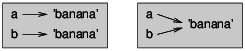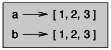# 1.10: Objects and Values

If we execute these assignment statements:

a = 'banana'
b = 'banana'


We know that a and b both refer to a string, but we don’t know whether they refer to the same string. There are two possible states, shown in Figure 10.10.1.Figure $$\PageIndex{1}$$: State diagram.

In one case, a and b refer to two different objects that have the same value. In the second case, they refer to the same object.

To check whether two variables refer to the same object, you can use the is operator.

>>> a = 'banana'
>>> b = 'banana'
>>> a is b
True


In this example, Python only created one string object, and both a and b refer to it.

But when you create two lists, you get two objects:

>>> a = [1, 2, 3]
>>> b = [1, 2, 3]
>>> a is b
False


So the state diagram looks like Figure 10.10.2.Figure $$\PageIndex{2}$$: State diagram.

In this case we would say that the two lists are equivalent, because they have the same elements, but not identical, because they are not the same object. If two objects are identical, they are also equivalent, but if they are equivalent, they are not necessarily identical.

Until now, we have been using “object” and “value” interchangeably, but it is more precise to say that an object has a value. If you execute [1,2,3], you get a list object whose value is a sequence of integers. If another list has the same elements, we say it has the same value, but it is not the same object.